Trigonometry : Determine Vertical Shifts

Example Questions

Example Question #1 : Determine Vertical Shifts

Let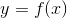be a function defined as follows: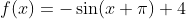The 4 in the function above affects what attribute of the graph of?

Amplitude

Vertical shift

Period

Phase shift

Vertical shift

Explanation:

The period of the function is indicated by the coefficient in front of; here the period is unchanged.

The amplitude of the function is given by the coefficient in front of the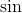; here the amplitude is -1.

The phase shift is given by the value being added or subtracted inside thefunction; here the shift isunits to the right.

The only unexamined attribute of the graph is the vertical shift, so 4 is the vertical shift of the graph. A vertical shift of 4 means that the entire graph of the function will be moved up four units (in the positive y-direction).

Example Question #2 : Determine Vertical Shifts

Letbe a function defined as follows: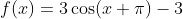What is the vertical shift in this function?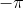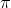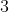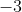Explanation:

The period of the function is indicated by the coefficient in front of; here the period is unchanged.

The amplitude of the function is given by the coefficient in front of the; here the amplitude is 3.

The phase shift is given by the value being added or subtracted inside the cosine function; here the shift isunits to the right.

The only unexamined attribute of the graph is the vertical shift, so -3 is the vertical shift of the graph. A vertical shift of -3 means that the entire graph of the function will be moved down three units (in the negative y-direction).

Example Question #121 : Trigonometric Functions And Graphs

The graph below shows a translated sine function. Which of the following functions could be shown by this graph?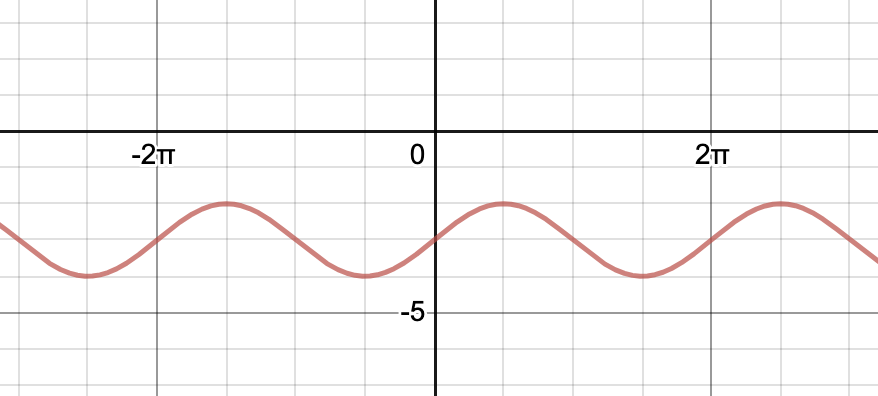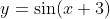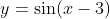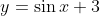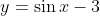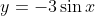Explanation:

A normal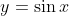graph has its y-intercept at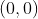. This graph has its y-intercept at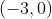. Therefore, the graph was shifted down three units. Therefore the function of this graph is.

Example Question #122 : Trigonometric Functions And Graphs

This graph shows a translated cosine function. Which of the following could be the equation of this graph?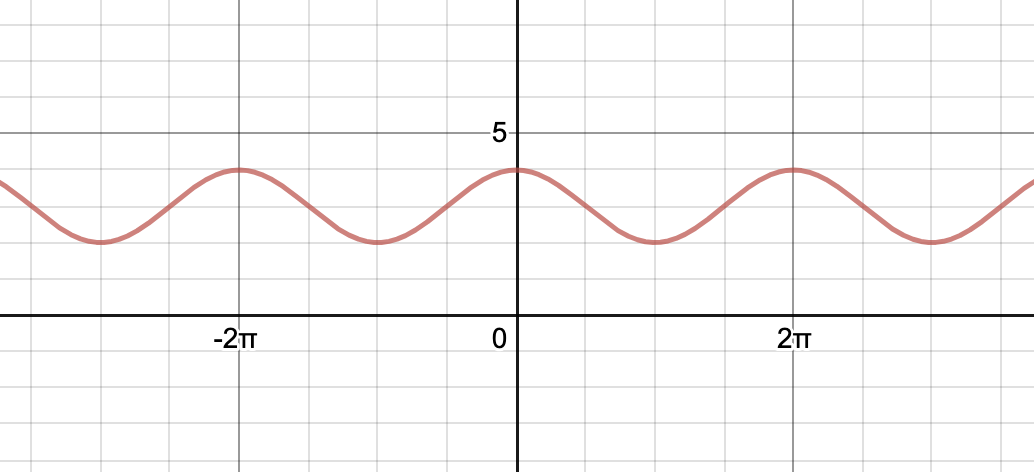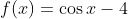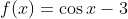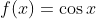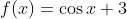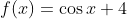Explanation:

The correct answer is. There are no sign changes with vertical shifts; in other words, when the function includes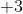, it directly translates to moving up three units. If you thought the answer was, you may have spotted the y-intercept at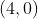and jumped to this answer. However, recall that the y-intercept of a regular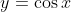function is at the point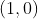. Beginning atand ending atcorresponds to a vertical shift of 3 units.

Example Question #5 : Determine Vertical Shifts

Consider the function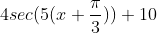.  What is the vertical shift of this function?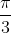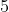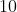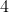Explanation:

The general form for the secant transformation equation is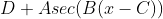.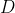represents the phase shift of the function.  When consideringwe see that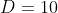, so our vertical shift isand we would shift this functionunits up from the original secant function’s graph.

Example Question #6 : Determine Vertical Shifts

Which of the following is the graph of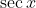with a vertical shift of?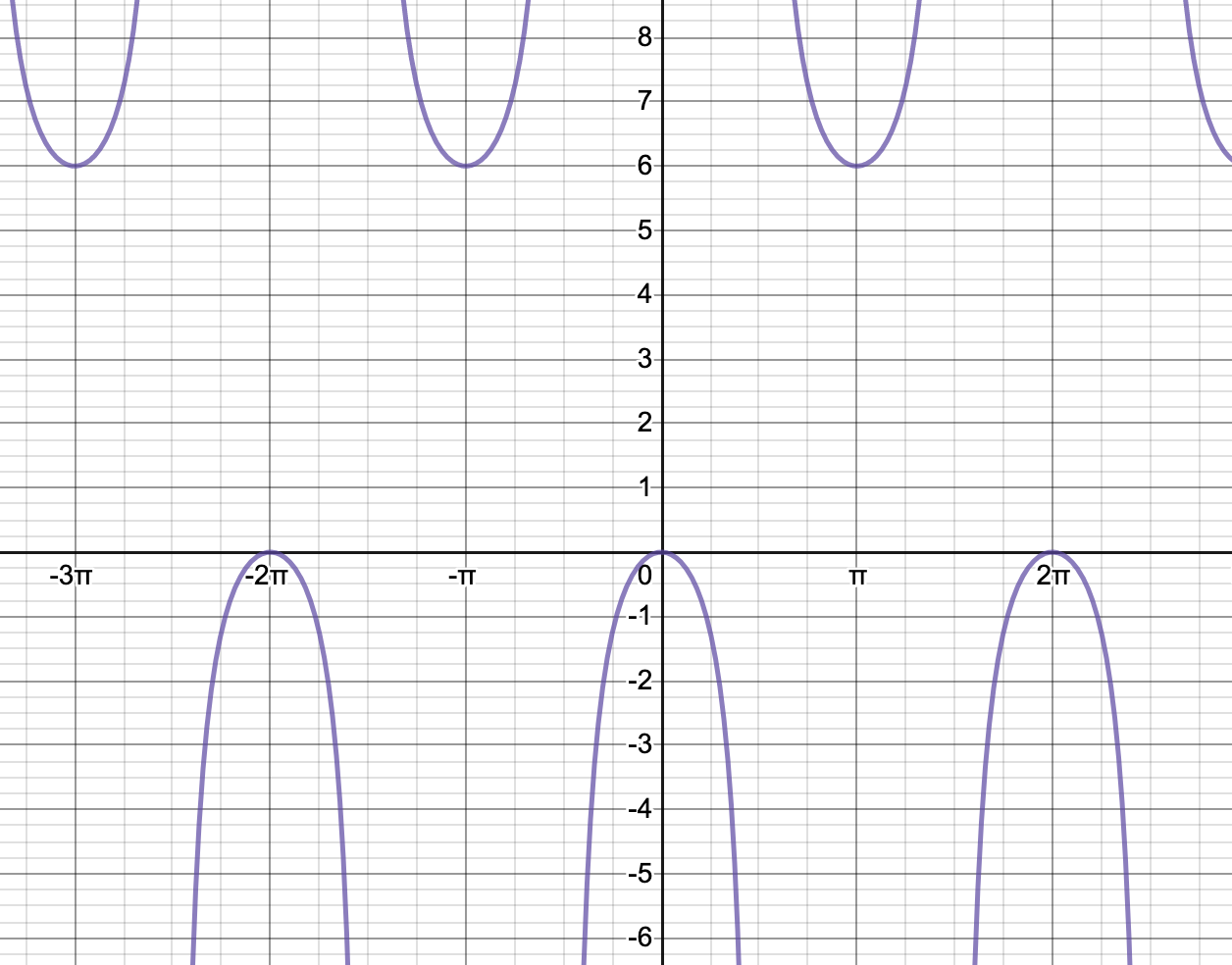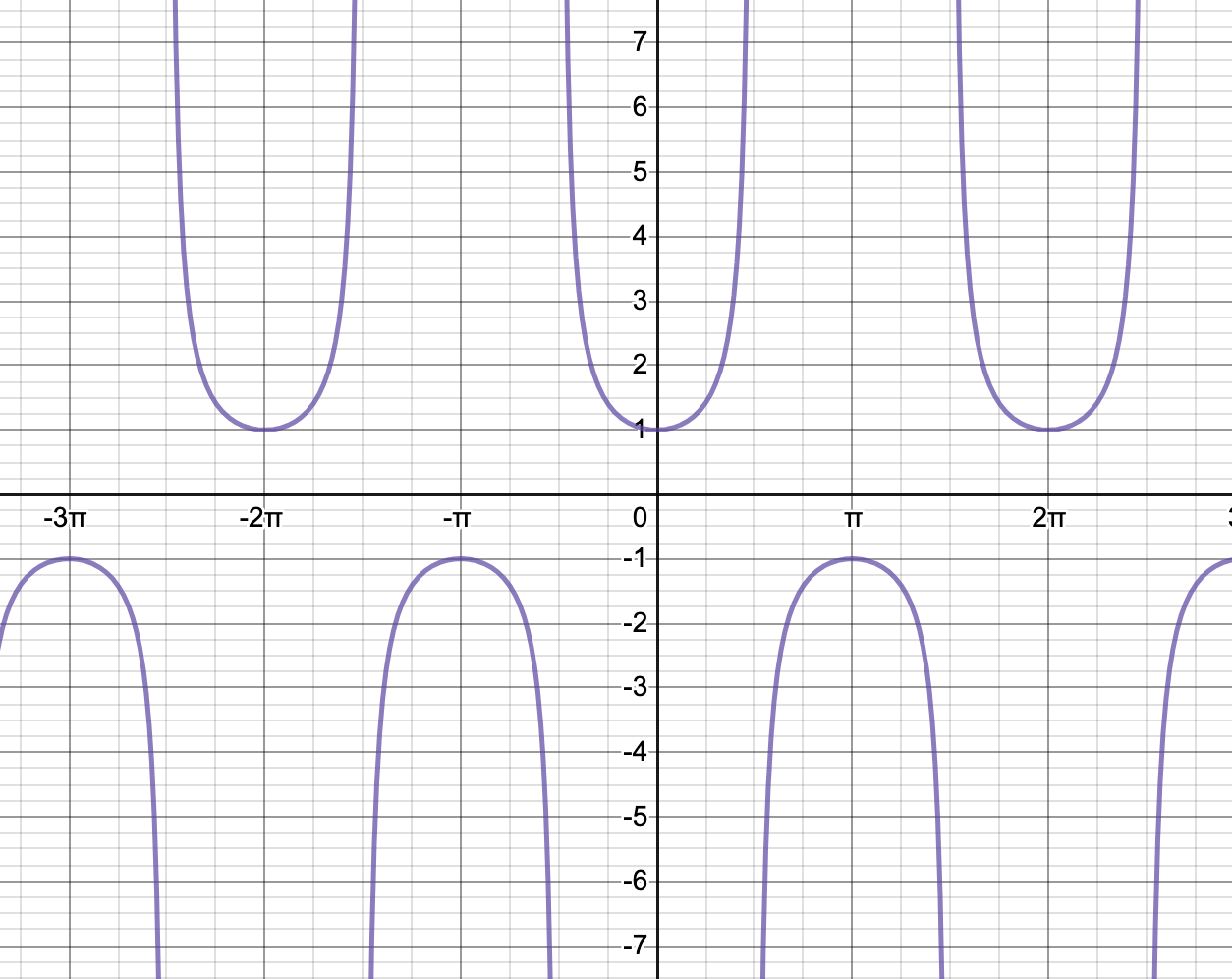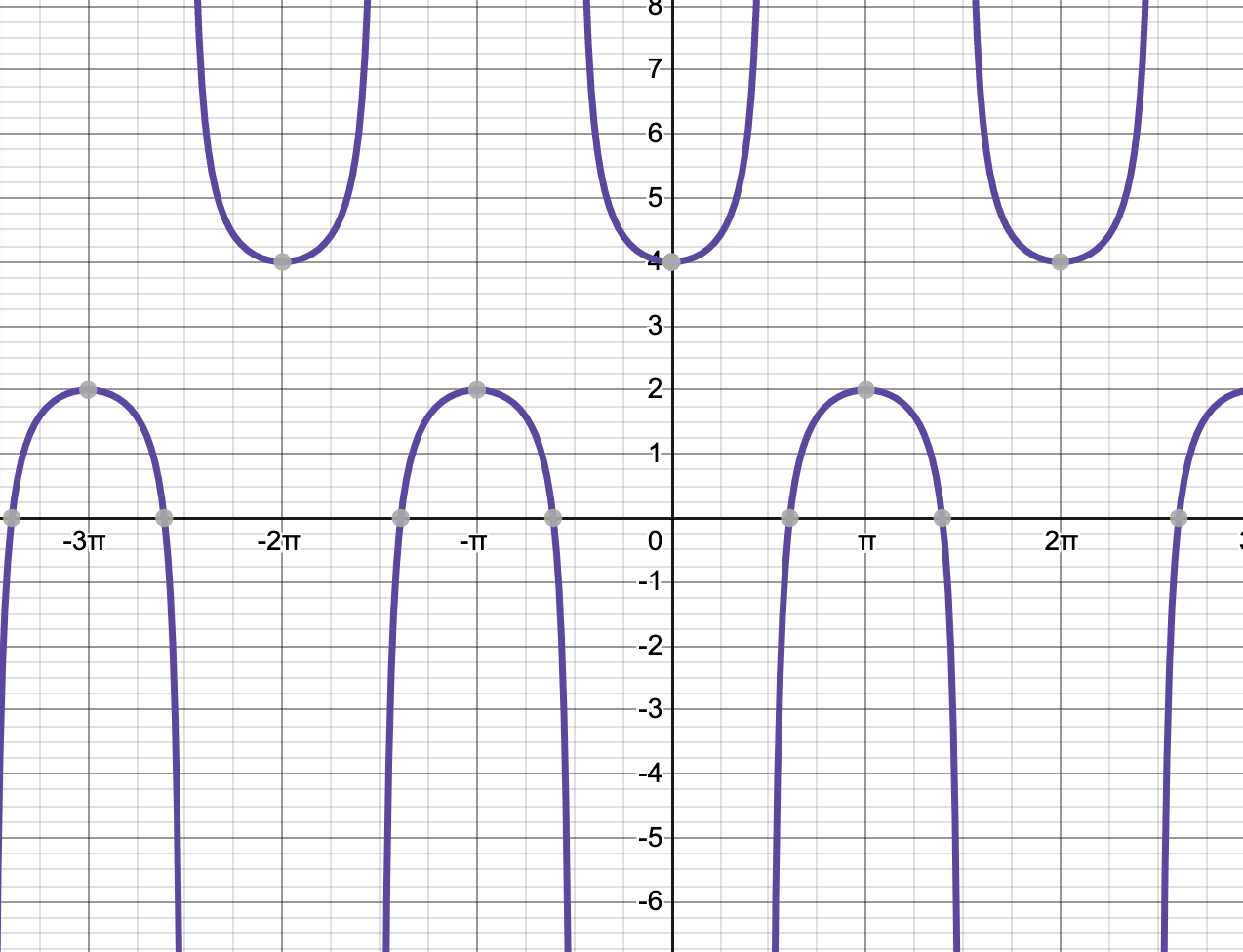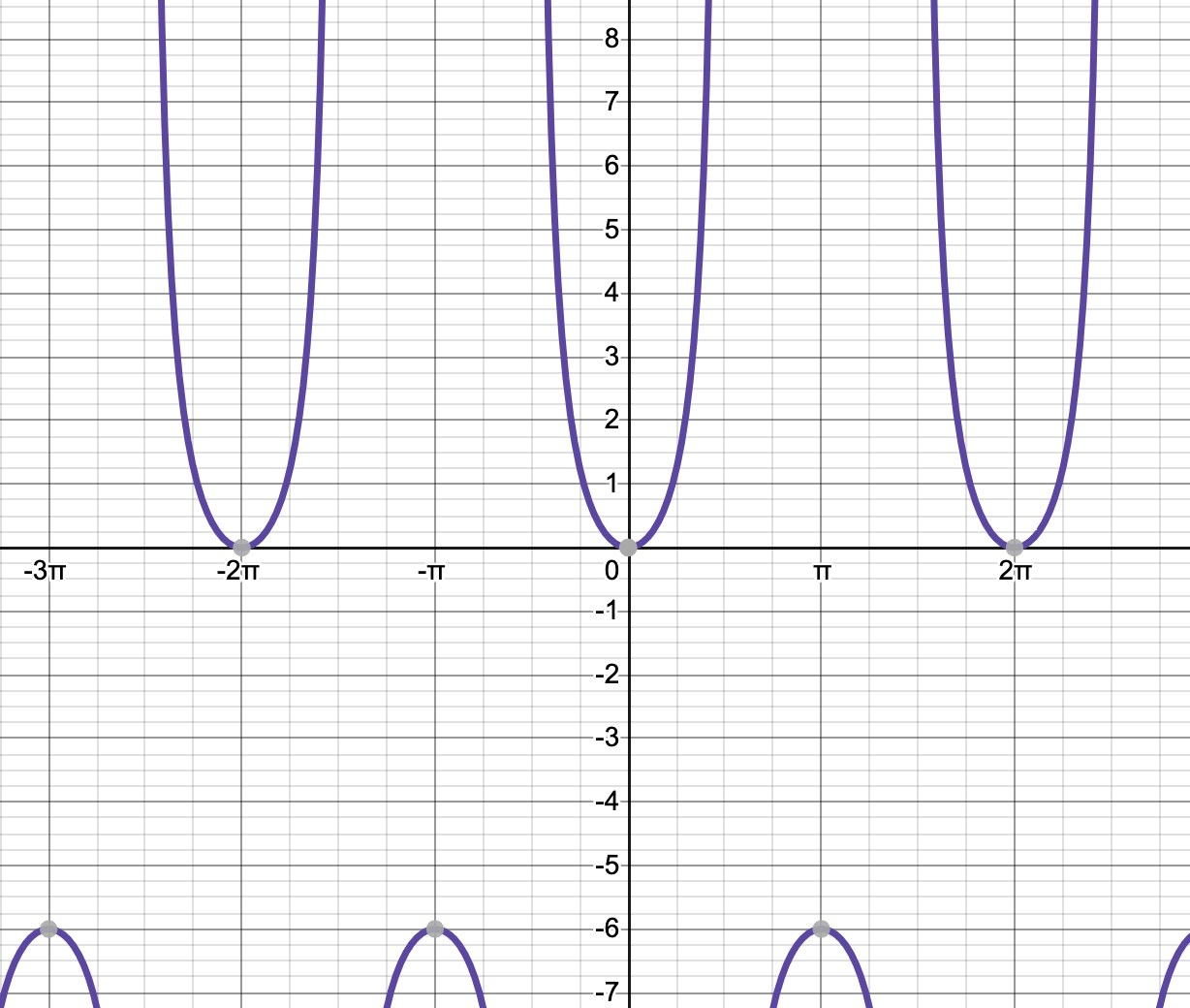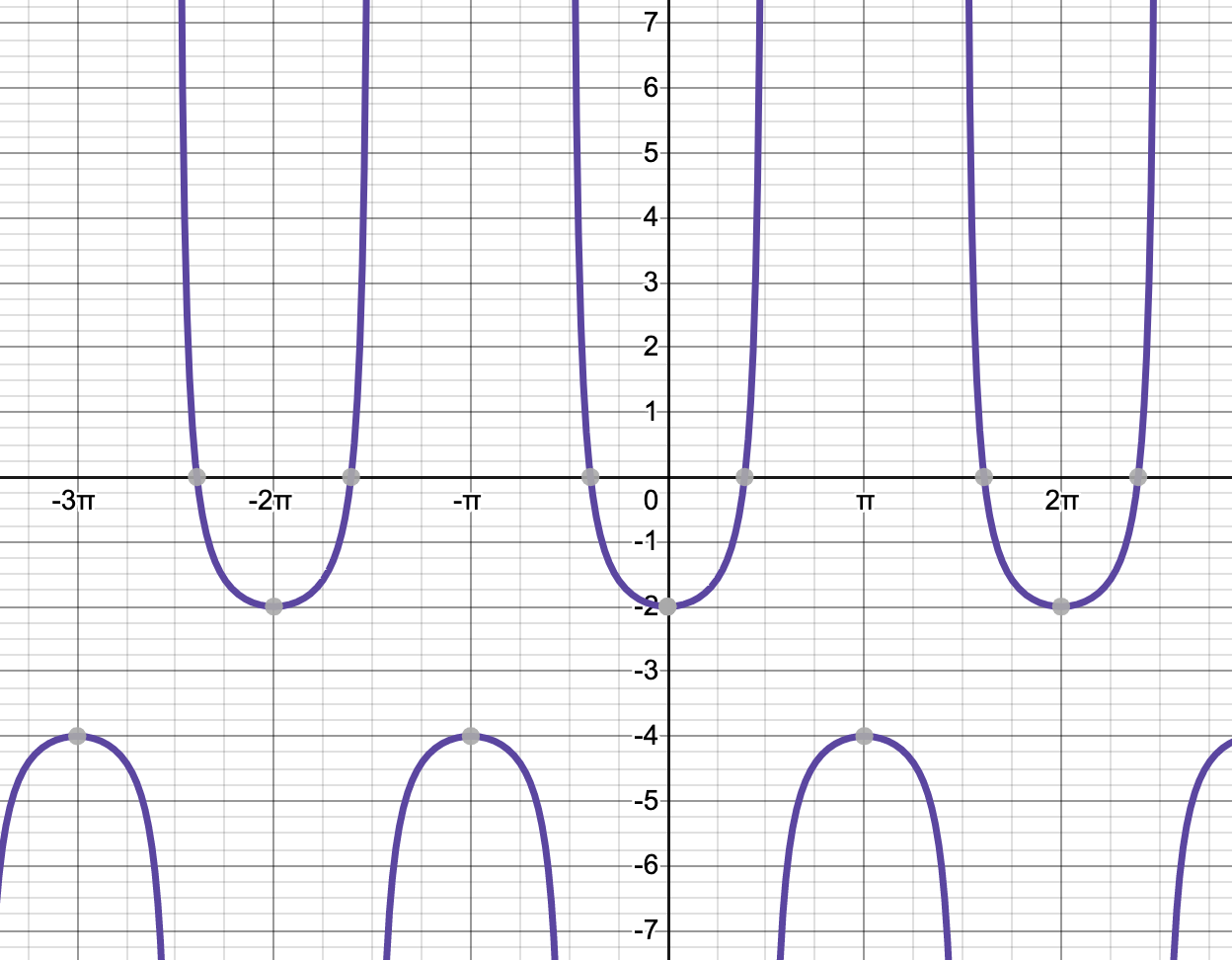Explanation:

The graph ofwith a vertical shift ofis shown below. This can also be expressed as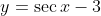.Here is a graph that shows both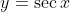and, so that you can see the "before" and "after." The original function is in blue and the translated function is in purple.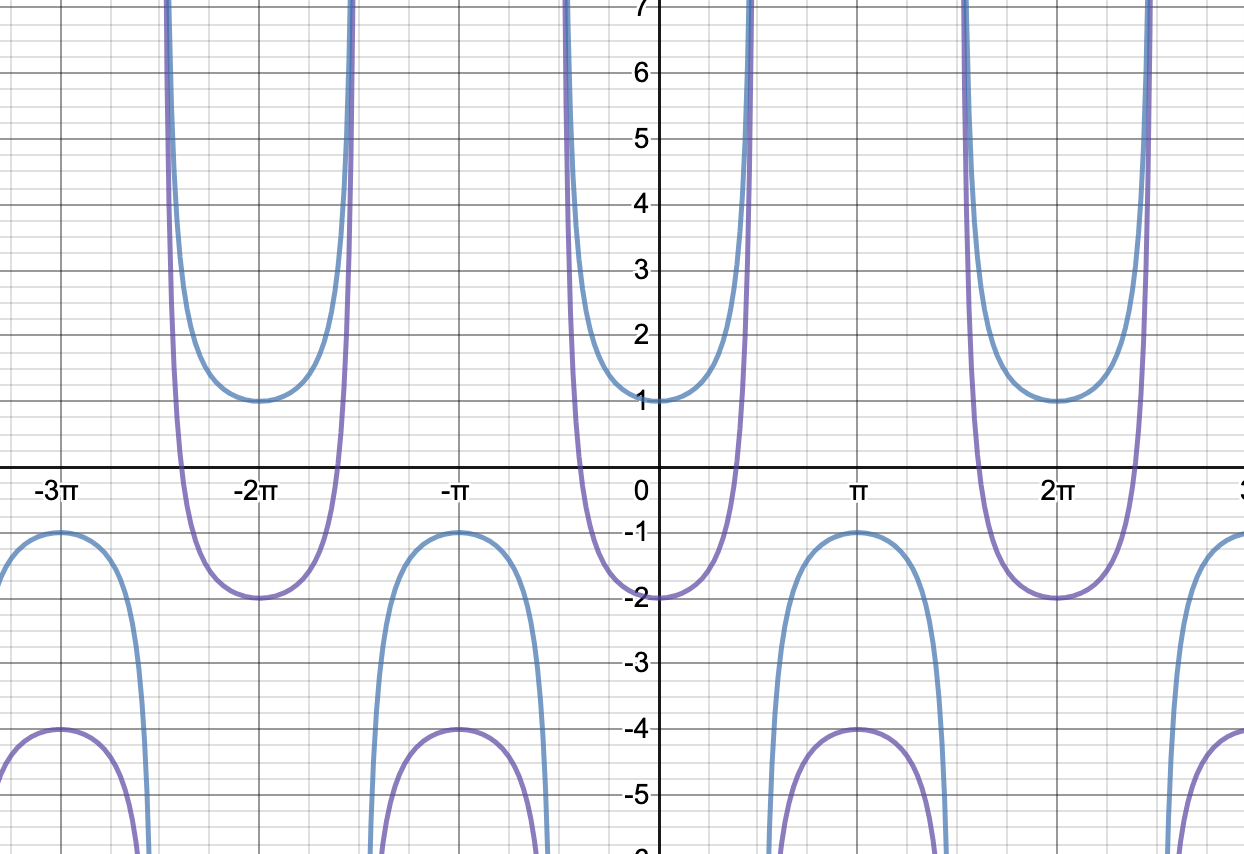The graphs of the incorrect answer choices are(no vertical shift applied),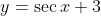(shifted upwards instead of downwards),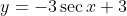(amplitude modified, and shifted upwards instead of downwards), and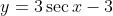(shifted downwards 3 units, but this is not the correct original graph of simplysince the amplitude was modified.)

Example Question #7 : Determine Vertical Shifts

Which of the following graphs shows one of the original six trigonometric functions with a vertical shift ofapplied?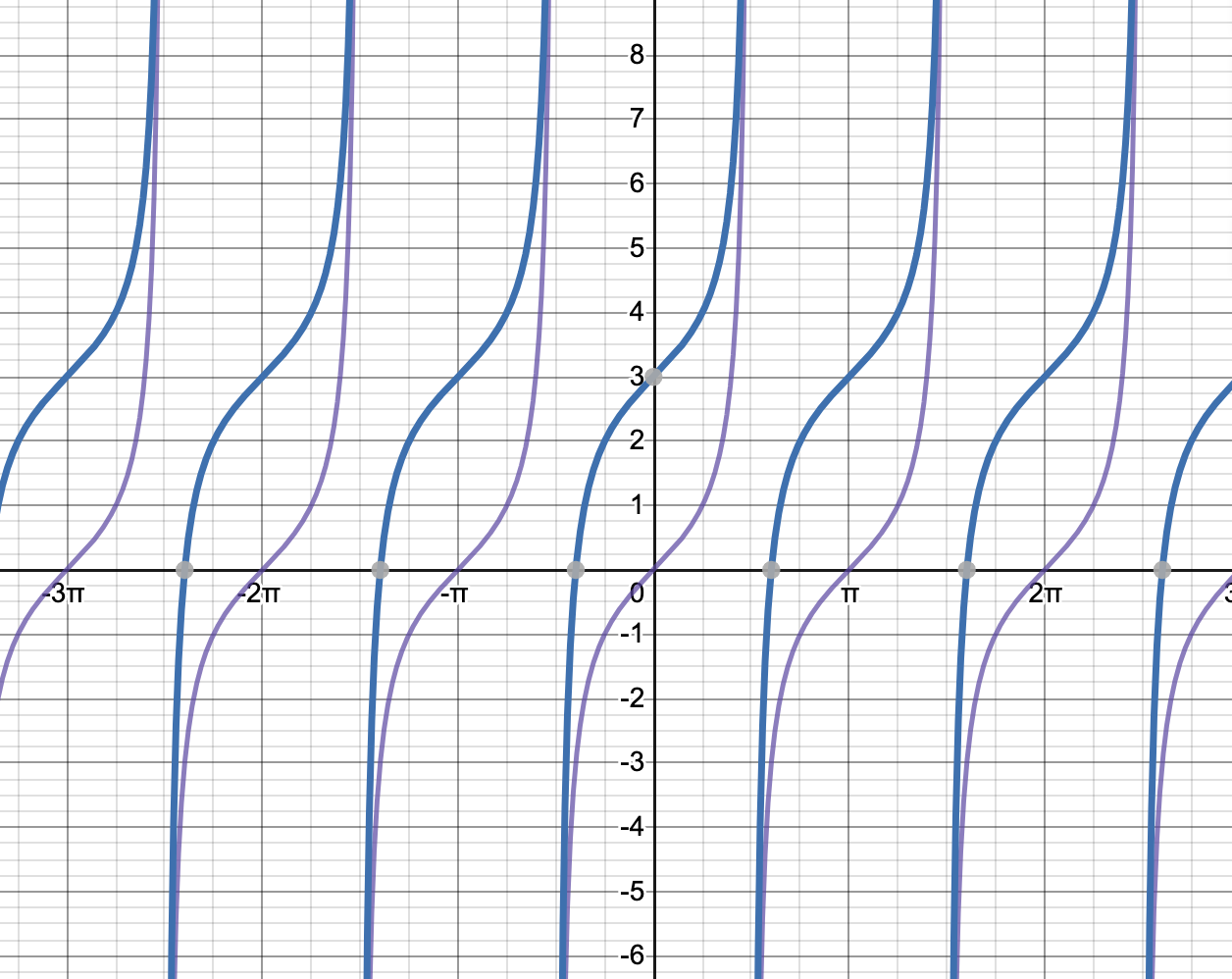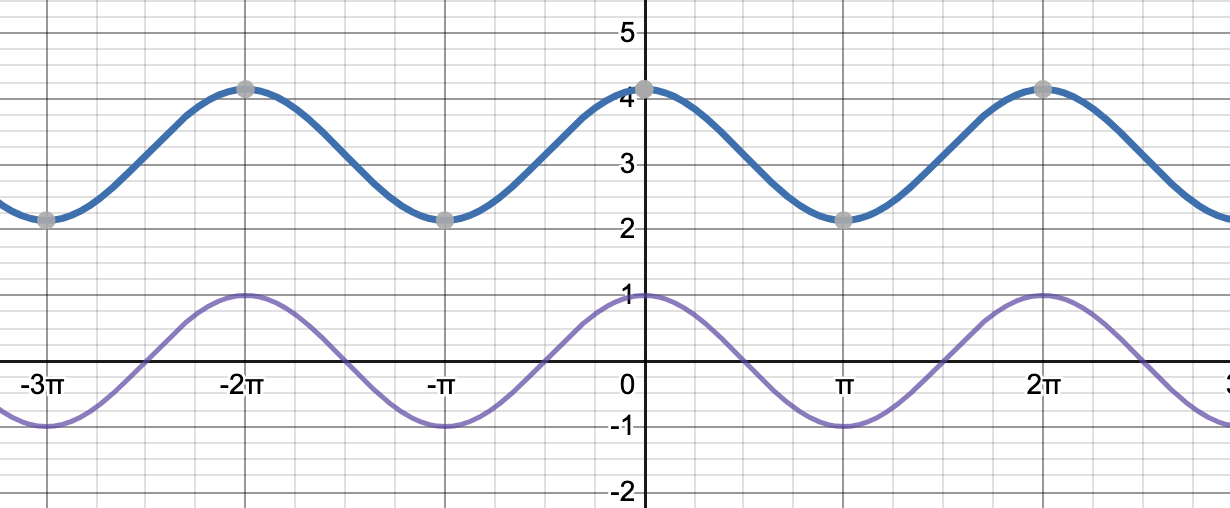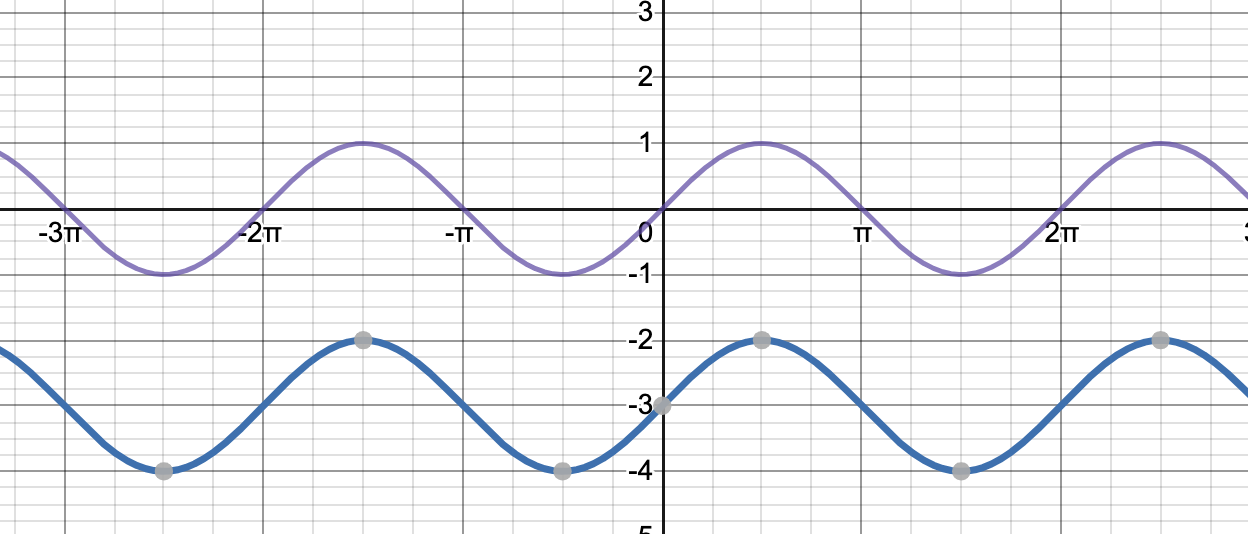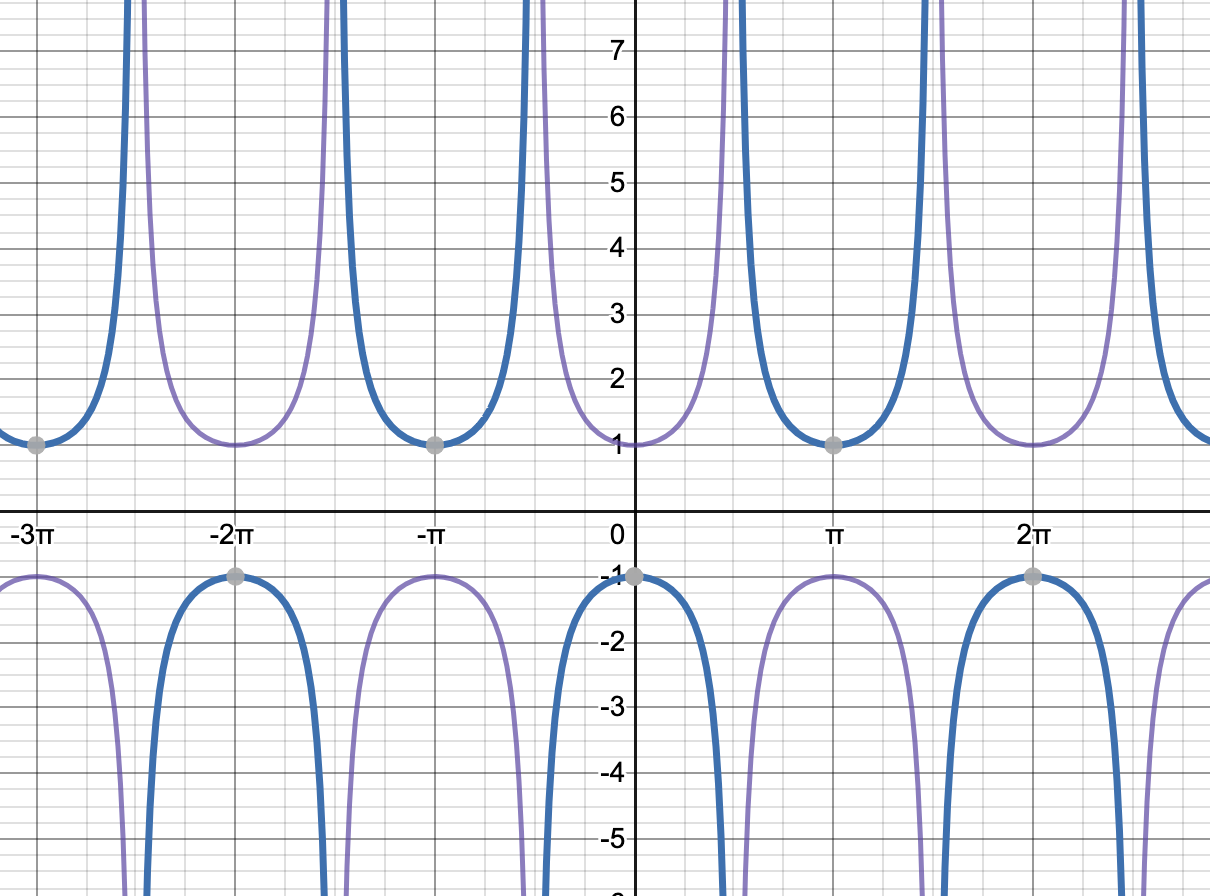We are looking for an answer choice that has one of the six trigonometric functions, as well as that function shifted up 3 units. The only answer choice that displays that is this graph of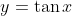(purple) and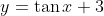(blue).The incorrect answers depictandand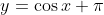, andand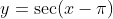.